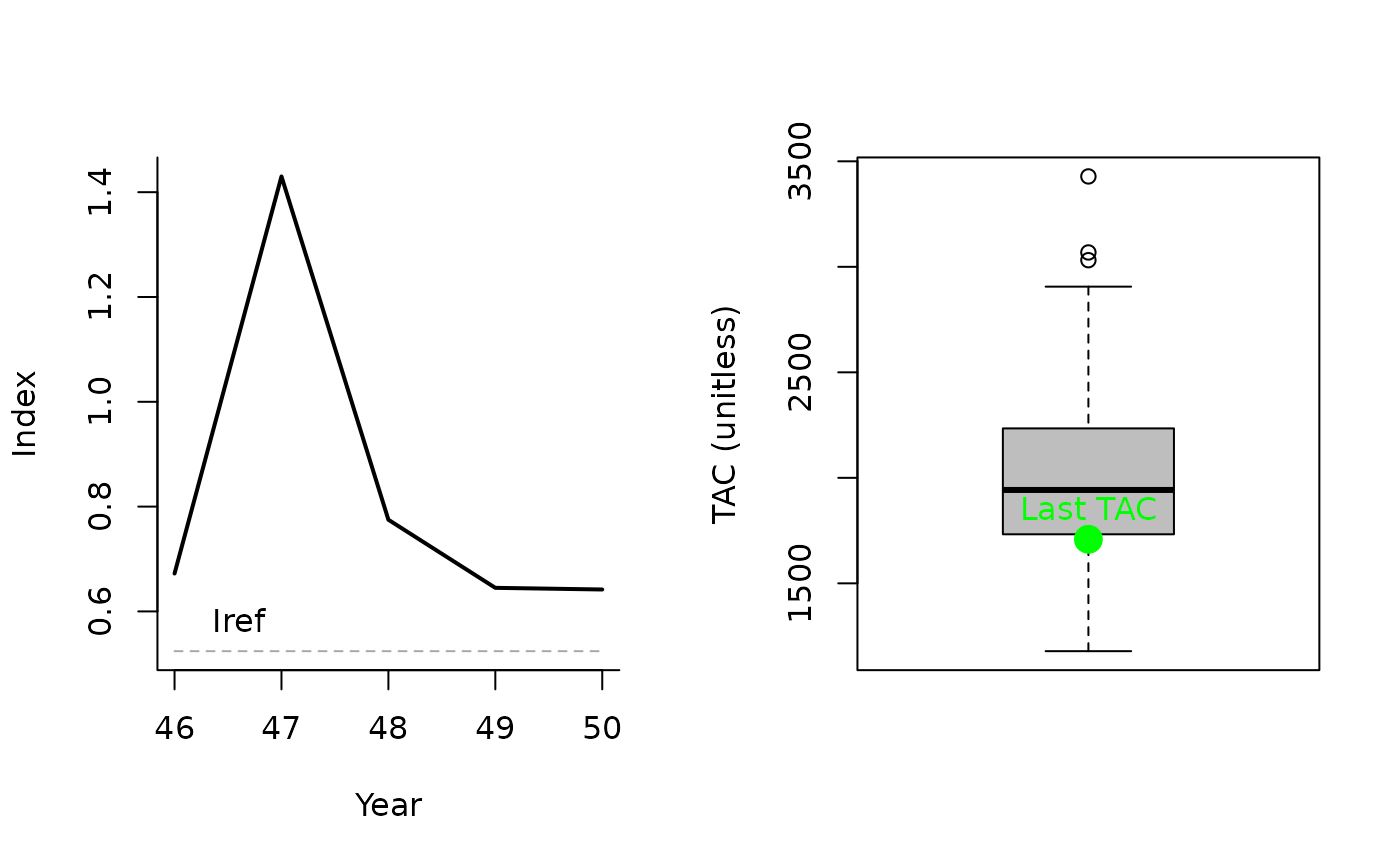An index target MP where the TAC is modified according to current index levels (mean index over last number of years determined by natural mortality (M)) relative to a target level.

ITM(x, Data, reps = 100, plot = FALSE)

## Arguments

x

A position in the data object

Data

A data object

reps

The number of stochastic samples of the MP recommendation(s)

plot

Logical. Show the plot?

## Value

An object of class Rec-class with the TAC slot populated with a numeric vector of length reps

## Details

The TAC is caluclated as: $$\textrm{TAC}_y = \textrm{TAC}_{y-1} \delta I$$ where $$\delta I$$ is the ratio of the mean index over $$4\frac{1}{M}^{1/4}$$ years to the reference index (Data@Iref).

The maximum fractional change in TAC is determined by $$mc$$, defined as $$mc = \textrm{max}\left(\frac{5 + 25M}{100}, 0.2\right)$$

## Required Data

See Data-class for information on the Data object

ITM: Ind, Iref, MPrec, Mort

## Rendered Equations

See Online Documentation for correctly rendered equations

T. Carruthers

## Examples

ITM(1, Data=MSEtool::SimulatedData, plot=TRUE)#> TAC (median)
#>     1938.764